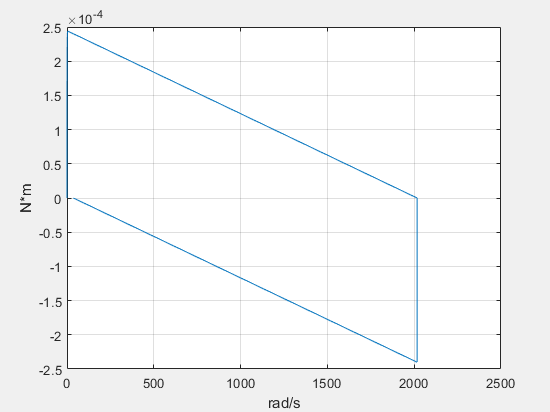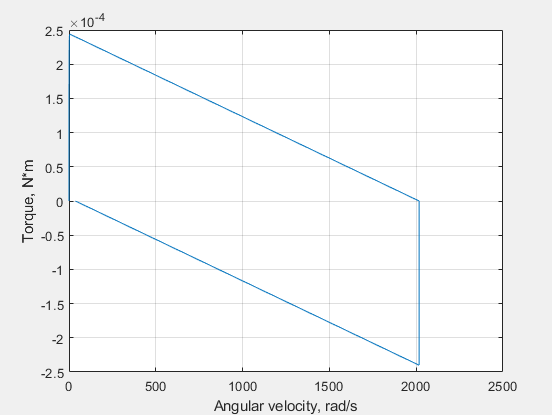# plotxy

Plot two series against each other

## Syntax

``fh = plotxy(x,y)``
``fh = plotxy(x,y,Name,Value)``

## Description

example

````fh = plotxy(x,y)` plots values of the simulation series `y` along the `y`-axis, with values of the simulation series `x` along the `x`-axis. `fh` is a cell array of handles to the resulting figures. `x` and `y`are `simscape.logging.Series` objects or homogeneous cell arrays of such objects. All series must have the same time vectors. If `x` and `y` are cell arrays, they must be of the same size, or one of them can be a scalar. ```

example

````fh = plotxy(x,y,Name,Value)` lets you customize the plot by using one or more `Name,Value` pair arguments. For example, specify '`time`' followed by a 1x2 vector, ```[start_time end_time]```, to plot only the data within this time range.```

## Examples

collapse all

Plot the motor torque series against its angular velocity series.

Open the Permanent Magnet DC Motor example model:

```openExample('simscape/PermanentMagnetDCMotorExample') ```

This example model has data logging enabled for the whole model, with the Workspace variable name parameter set to `simlog_PermanentMagnetDCMotor`.

Simulate the model to log the simulation data:

`sim('PermanentMagnetDCMotor');`

Plot the motor torque against its angular velocity:

```simscape.logging.plotxy(simlog_PermanentMagnetDCMotor.DC_Motor.Rotational_Electromechanical_Converter.R.w.series,... simlog_PermanentMagnetDCMotor.DC_Motor.Inertia.t.series)```When you plot the series against each other, the default plot displays only the unit names along each axis. To add the axis names, use name-value pair arguments.

```simscape.logging.plotxy(simlog_PermanentMagnetDCMotor.DC_Motor.Rotational_Electromechanical_Converter.R.w.series,... simlog_PermanentMagnetDCMotor.DC_Motor.Inertia.t.series,'xname','Angular velocity','yname','Torque')```## Input Arguments

collapse all

Simulation data to plot along the x-axis, specified as a `simscape.logging.Series` object or a homogeneous cell array of such objects. `x` must include a full identifier path to the series, starting with the workspace log variable name. If `x` and `y` are cell arrays, they must be of the same size, or one of them can be a scalar. All series must have the same time vectors.

Simulation data to plot along the y-axis, specified as a `simscape.logging.Series` object or a homogeneous cell array of such objects. `y` must include a full identifier path to the series, starting with the workspace log variable name. If `x` and `y` are cell arrays, they must be of the same size, or one of them can be a scalar. All series must have the same time vectors.

### Name-Value Arguments

Specify optional pairs of arguments as `Name1=Value1,...,NameN=ValueN`, where `Name` is the argument name and `Value` is the corresponding value. Name-value arguments must appear after other arguments, but the order of the pairs does not matter.

Before R2021a, use commas to separate each name and value, and enclose `Name` in quotes.

Example: ```fh = plotxy(simlog.TS.C.v.series,simlog.TS.R.v.series,'xunit','mm/s','yunit','mm/s')``` plots velocities of ports C and R of the Translational Spring block `TS` against each other, in `mm/s`.

Time range for plotting the data, specified as the comma-separated pair consisting of '`time`' and a 1x2 vector, ```[start_time end_time]```, in seconds.

`[]` plots all data.

Name of the plot x-axis, specified as the comma-separated pair consisting of '`xname`' and a character vector, string scalar, or a cell array of character vectors or string scalars. The number of elements in the cell array must be same as the number of elements of `x`.

Name of the plot y-axis, specified as the comma-separated pair consisting of '`yname`' and a character vector, string scalar, or a cell array of character vectors or string scalars. The number of elements in the cell array must be same as the number of elements of `y`.

Unit for plotting the data along the x-axis, specified as the comma-separated pair consisting of '`xunit`' and a unit name, or a cell array of unit names. Unit names must appear inside single quotes (`''`) or double quotes (`""`). Specified units must be commensurate with the units of the series values.

Unit for plotting the data along the y-axis, specified as the comma-separated pair consisting of '`xunit`' and a unit name, or a cell array of unit names. Unit names must appear inside single quotes (`''`) or double quotes (`""`). Specified units must be commensurate with the units of the series values.

## Output Arguments

collapse all

Handles to the resulting plot figure windows, one for each `y` versus `x` plot generated, returned as a cell array.

## Version History

Introduced in R2010b